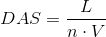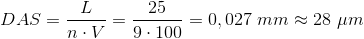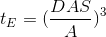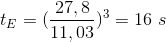## Dendrite arm spacing

Dendrite arm spacing is called the distance between the dendrites secondary arms (Fig. 1) as is illustrated by the micrograph. In textbooks, the dendrite arm spacing als often referred to as DAS or as SDAS (Secondary Dendrite Arms Spacing).To determine the dendrite arm spacing, the total spacing from the first to the last arm needs to be determined, i.e. the measuring length L in millimeters (well developed and parallel dendrite arms in the micrograph should be selected). By considering the micrographmagnification V, it is divided by the number n of exinsting dendrite arms in this area and, according to eq. 1 , converted to the unit µm. After repeated measurements in different areas an average value of the results is calculated.

Eq. 1:In the following example in Fig. 2, nine dendrite arms are measured. In the micrograph with a magnification of V = 100: 1, the measuring length L of 25mm is calculated. The dendrite arm spacing can be calculated on eq. 2 as follows:

Eq. 2:The dedrite arm spacing is the bigger, the slower the material solidifies (i.e. fewer but thicker dendrite arms at the same measuring length develop). The solidification time tE is authoritative for the dendrite arm spacing whereas in general the following principle (eq. 3) applies:

Eq. 3:In eq. 3, A represents a material-dependent constant. Regarding aluminum casting alloys, for the type Al Si7Mg this is 11.03 und for Al Cu4Ti this is 12.4. When using alloys for gravity die casting (see also aluminum gravity die casting alloys), shorter solidification times and smaller dendrite arm spacing are present compared to sand casting. In eq. 3, tE is inserted in seconds and DAS in µm. For the example and the calculated arm spacing of 30 µm, regarding the Al Si7Mg alloy a solidification time of 16 sec could be calculated on the basis of eq. 4:

eq. 4:In general, aluminum die casting alloys have improved mechanical properties if the solidification time is low (smaller averaged DAS). The faster the materials solidifies, the higher are tensile strength, 0.2 yield strength and elongation. This relation is illustrated by Fig. 3. The conclusion is that the application of cooling chills for sand casting has a positive effect on mechanical properties if sufficient feeding of the casting parts occurs. This effect, including an increase in tensile strength, a 0.2 percent yield strength and elongation, i.e. an increase in strengths and ductility, otherwise can only be achieved through grain refinement to a very small extent.In case of cast steel, the dendrite arm spacing influences these properties to a larger extent than the primary grain size, the notched-bar impact strength and the elongation at failure decrease the higher the dendrite arm spacing is. This effect can clearly be seen with high-tensile heat retreatable steel (Rm > 1500 MPa).

Modern image analyzing systems enable calibrated images so that the DAS can immediately be measures from the image, as shown in Fig. 4. For excluding single coarsened or minimized dendrite trunks, the median and not the average should be evaluated (Table 1).